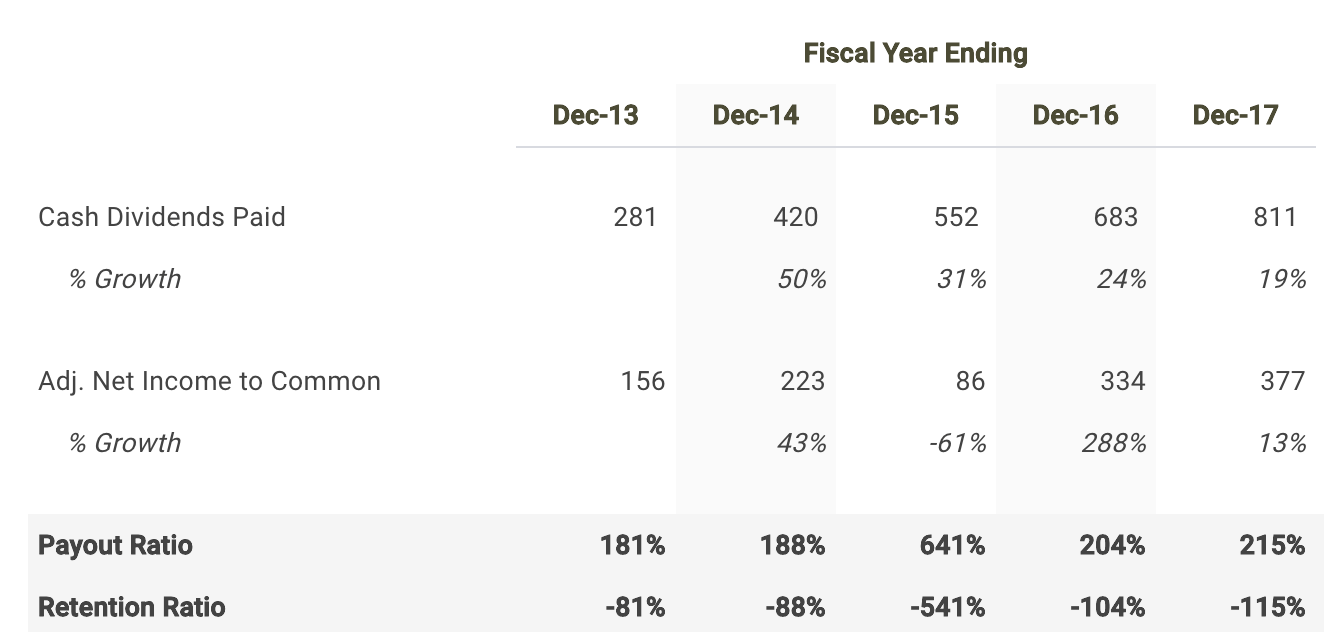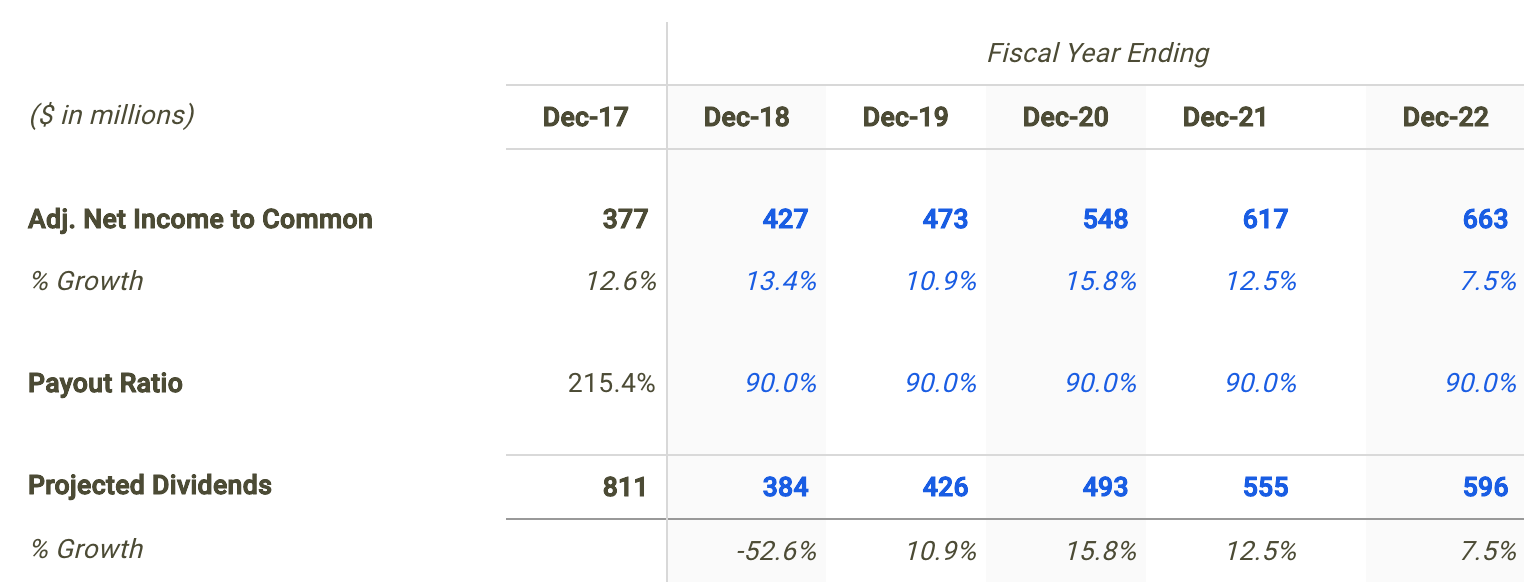Western Gas Equity Partners (NYSE: WGP) has distributed 224.4% of its profits in the form of dividends over the last twelve months making the stock a prime candidate to be valued using a dividend discount analysis. Operating as an energy company paying a dividend yield of 6.3%, could the mid-cap stock be trading at an attractive valuation? Let’s take a look at the future dividend potential of WGP to see if there is any catalyst for a price change.

##### Application Of The Dividend Discount Model

The Dividend Discount Model (DDM) is a dividend-based valuation model that estimates the present value of a stock based on assumptions about its future dividend performance.

But before discussing the assumptions used in my dividend discount model for WGP, it’s first helpful to determine if this is actually an appropriate technique to be used when estimating its fair value. Many analysts find it difficult when trying to figure out the correct valuation methodology for a given company or are biased towards one specific approach. This is often a mistake which can negatively impact investment decisions and result in trading losses or missed opportunities. No two companies are the same and every business consists of unique characteristics that may require you to adjust your analysis.

Understanding leverage trends is the first step when determining what valuation analyses are relevant for a given company. When a company’s leverage doesn’t fluctuate or is expected to remain stable over time, then an equity valuation model (e.g. equity DCF, DDM) will be the most appropriate valuation technique. The reason for this is because when leverage is stable, interest expense on debt can typically be projected with much more reliability.

How do we check if a company’s leverage has been fluctuating or is expected to do so? This isn’t always straightforward but checking recent debt ratio trends can be a good indicator. WGP’s debt to equity ratio has been relatively consistent over last few years with a range spanning only 15.1%. This suggests that an equity valuation model is a suitable technique when valuing the company’s shares. Now does it make sense to use a dividend discount model knowing that an equity valuation technique is an appropriate methodology?

The next step is to determine if WGP pays a dividend and if so, is its payout ratio relatively high (typically above 70%)? The table below provides this information in more detail.Source: WGP dividend discount model

WGP distributed a total of \$811 million in cash dividends to shareholders in its most recent fiscal year Dec-17 which represented a payout ratio of 215.4%. It appears that the company meets both criteria. Therefore, it is fitting to apply a dividend discount model when calculating the intrinsic value of WGP.

##### Projecting The Future Dividends Of WGP

The first step in building a dividend discount model is to forecast net income since forecasting dividends directly can be difficult. So let’s create a net income forecast for the next five years and use that as the basis for projecting future dividends.

Finbox.io applies consensus Wall Street estimates for the net income forecast when available. For the next fiscal year 2018, profits are expected to increase 13.4%, then grow 10.9% in 2019 and rise 15.8% in 2020.Source: WGP Projected Net Income Growth

I use the projections above to serve as the basis for my dividend projections. The next step is to forecast the company’s payout ratio. In my estimates shown in the table below, I select a 90.0% payout ratio in 2018 and hold it steady there throughout the remainder of my projection period.Source: WGP dividend discount model

##### Calculating WGP’s Intrinsic Value

Finally, we can now calculate WGP’s intrinsic value by present valuing its forecasted dividends. Note that we apply the company’s cost of equity to discount the future dividends since these payments are made to common shareholders or equity owners. I used finbox.io’s Weighted Average Cost of Capital (WACC) model to help arrive at an estimate for the company’s cost of equity.

I determined a reasonable cost of equity for WGP to be 11.9% at the midpoint. An updated cost of capital analysis using real-time data can be found at finbox.io’s WGP WACC model page.My dividend forecast and cost of capital assumptions imply a fair value per share for WGP of \$28.36, -20.8% below its current stock price of \$35.69. Therefore, WGP appears to be an overvalued stock and not necessarily a good entry point opportunity.

##### Conclusion: Dividends Don’t Support Stock Price

Finding the true value of a company can sometimes be difficult but determining an appropriate valuation methodology should not be. Knowing when and when not to use the dividend discount model will help in your investment decision making process.

But it is important to note that a dividend discount model will inherently undervalue a company’s stock. This is typically the result of the payout ratio assumption being less than 100% implying some cash leakage. In reality, this excess retained cash is usually paid out to shareholders as special dividends or to make up for cash shortfalls for future dividends during economic downturns.

This helps explain, at least partially, why WGP looks like an overvalued stock based on this approach. Nevertheless, a dividend discount analysis is still a helpful tool in combination with the understanding that it calculates a conservative intrinsic value estimate. Meaning an extremely attractive opportunity would be a stock where (1) a dividend discount model is appropriate and (2) shares looks undervalued based on its future dividends.

While a dividend discount analysis on its own is not necessarily indicative of a stock’s intrinsic value, it does provide helpful insights. I recommend that investors continue their research on WGP to gain a better understanding of all the factors driving its share price.

As of this writing, I did not hold a position in any of the aforementioned securities and this is not a buy or sell recommendation on any security mentioned.

Expertise: financial technology, analyzing market trends. Brian is a founder at finbox.io, where he’s focused on building tools that make it faster and easier for investors to research stock fundamentals. Brian’s background is in physics & computer science and previously worked as a software engineer at GE Healthcare. He enjoys applying his expertise in technology to help find market trends that impact investors. Brian can be reached at brian@finbox.io or at +1 (516) 778-6257.

This site uses Akismet to reduce spam. Learn how your comment data is processed.